2018-08-18 18:32:45 fengziyihang 阅读数 1618
• ###### MATLAB图像处理

全面系统的学习MATLAB在图像处理中的应用

19867 人正在学习 去看看 魏伟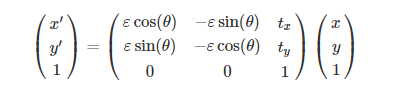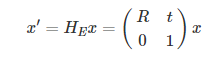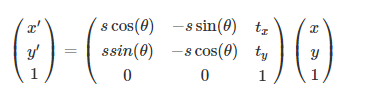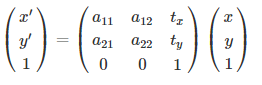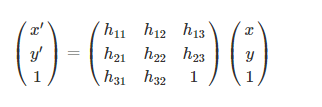2019-04-16 21:17:13 kuweicai 阅读数 655
• ###### MATLAB图像处理

全面系统的学习MATLAB在图像处理中的应用

19867 人正在学习 去看看 魏伟

## 2. 平移（Translation）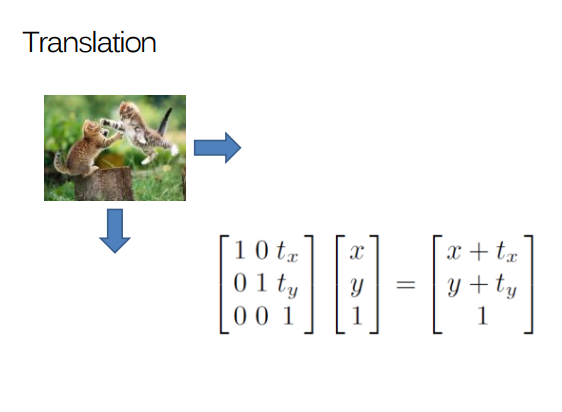## 3. 相似变换（Similarity transformations）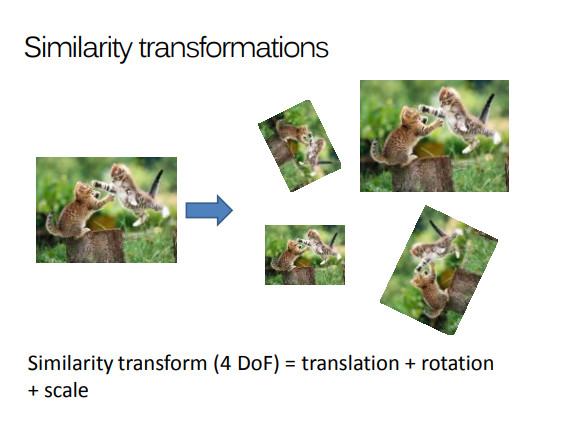## 3. 旋转（Rotate）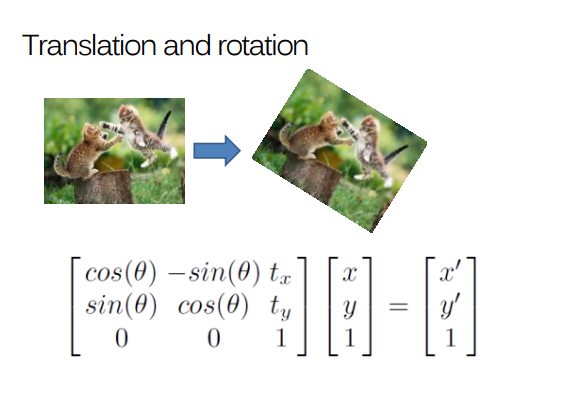## 4 长宽比（Aspect Ratio）调整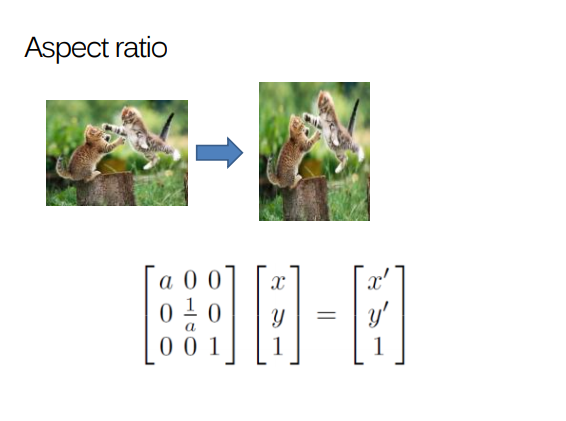## 5. 剪切（Shear）映射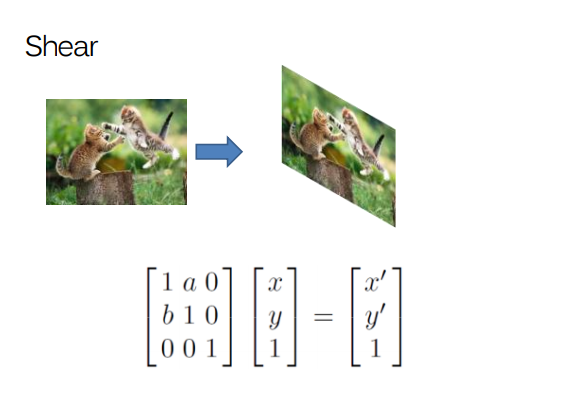## 6. 仿射变换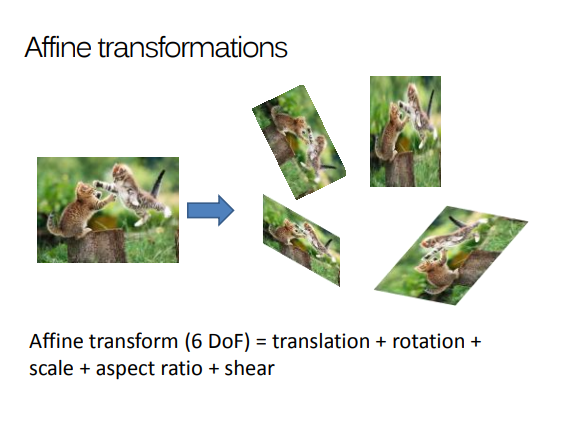$\left[ \begin{matrix} a & b & c \\ d & e & f \\ 0 & 0 & 1 \end{matrix} \right] \left[ \begin{matrix} x \\ y \\ 1 \end{matrix} \right] = \left[ \begin{matrix} x\prime \\ y \prime\\ 1 \end{matrix} \right]$

## 7. 单应性变换（Homogeneous Transformation）/ 透视变换（Perspective Transformation）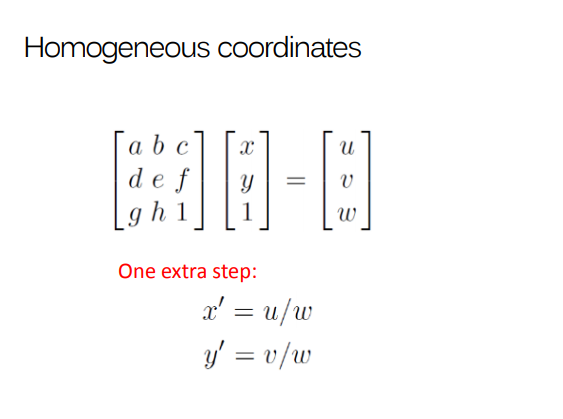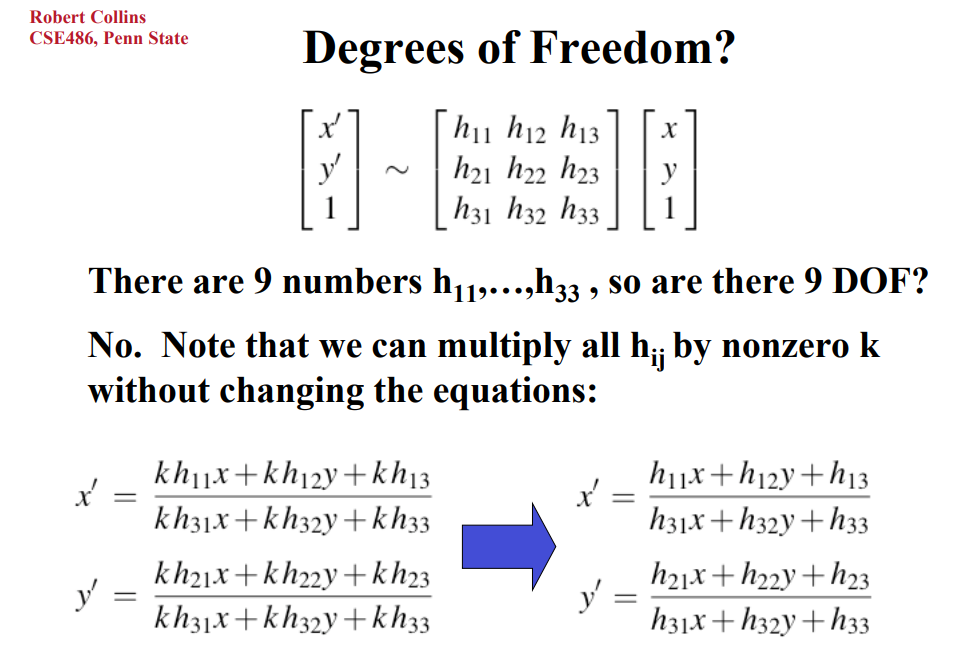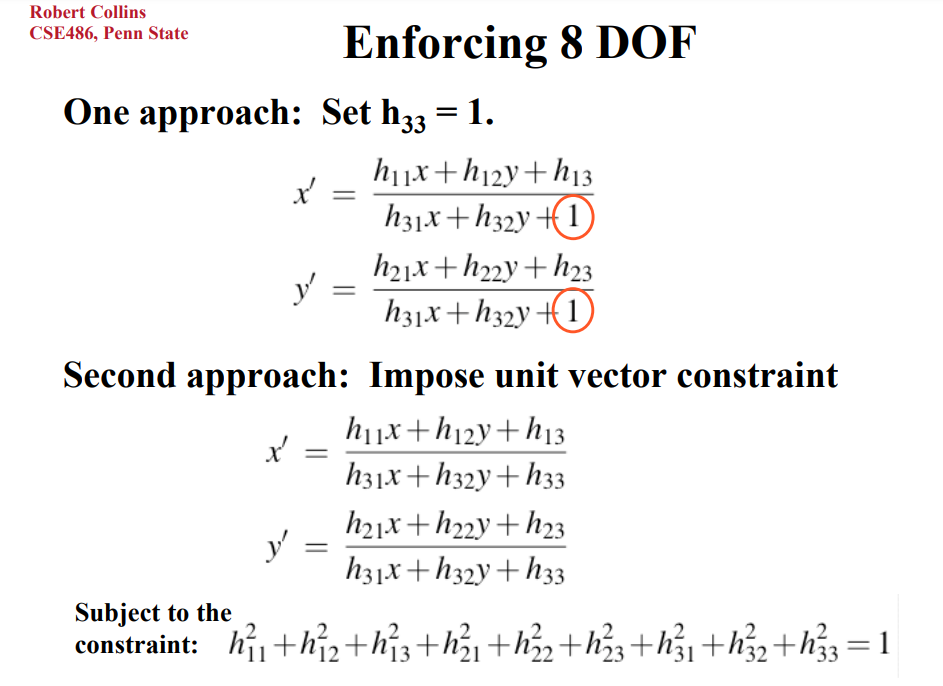## 参考

2019-06-18 20:50:50 lz867422770 阅读数 853
• ###### MATLAB图像处理

全面系统的学习MATLAB在图像处理中的应用

19867 人正在学习 去看看 魏伟

## 一. 前言

1. 最近在做身份证OCR项目中，需要对倾斜扭曲的图像做矫正，透视变换正可以解决这个问题，在这里记录对变换方法的理解。

2. 本文主要介绍一下仿射变换和透视变换的原理，特点以及其在opencv中实现的一些注意点。

3. 首先看下透视变换后的例图：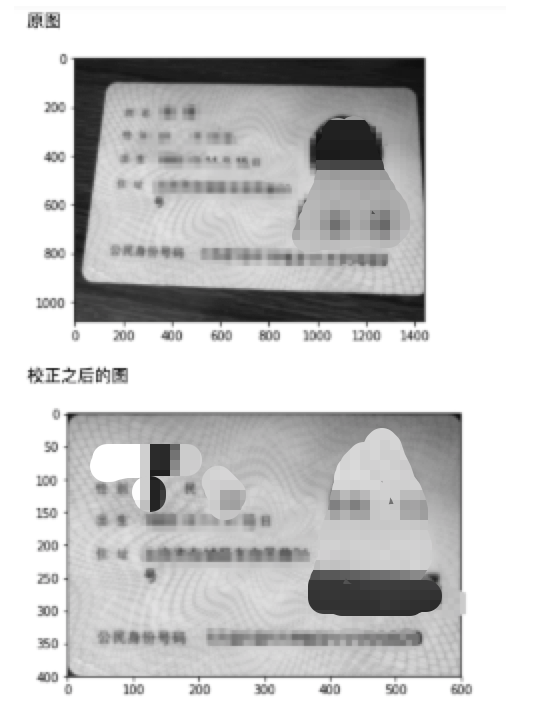## 二. 从仿射变换说起

1. 定义：仿射变换（Affine Transformation或 Affine Map），又称为仿射映射，是指在几何中，图像进行从一个向量空间进行一次线性变换和一次平移，变换为到另一个向量空间的过程。

2. 特性：仿射变换保持了二维图形的平直性和平行性。平直性：变换是直线的，变换后还是直线；平行性：二维图形之间的相对位置关系保持不变。

3. 公式：如图所示其中，

• M表示变换矩阵，它包括线性变换矩阵A和平移矩阵B；
• [x, y]T代表原始图像矩阵，是一个二维数组，x代表像素的横坐标，y代表像素的纵坐标；
• T代表经过仿射变换后的图像矩阵。

4. 理解：

• 在几何中，任何变换都可以以矩阵乘法（线性变换）的形式表示，图像中的平移操作可以以矢量加法（平移）的形式表示；

• 一个任意的仿射变换都可以表示为乘以一个矩阵再加上一个向量的形式；[x, y]T代表原始图像矩阵，是一个二维数组，x代表像素的横坐标，y代表像素的纵坐标；
• 如果线性变换矩阵A=[[1, 0], [0,1]]，那么A点乘矩阵[x, y]T 就不会改变[x, y]T的信息，此时，仿射变换就变成了平移操作，移动的行列值就是矩阵B中的元素值；
• 如果线性矩阵A=[[cosθ, -sinθ], [sinθ, cosθ]]，那么线性变换就变成了图片的旋转操作，旋转角度θ。矩阵B代表旋转中心的坐标便宜量。两个矩阵的计算方式如下图：5. 应用：

## 三. 再说透视变换

有了仿射变换的理解，下面说透视变换就简单了，因为它是仿射变换的一种非线性扩展。

1. 定义：透视变换(Perspective Transformation)就是将图片投影到一个新的视平面，也称为投影映射。

2. 特性：相对仿射变换来说，改变了直线之间的平行关系。

3. 直接看公式：通用的变换公式如下其中，

• u, v代表原始图片坐标，x, y为经过透视变换的图片坐标;
• 变换矩阵为3*3，它可以拆分为四个部分
• [a11, a12, a21, a22]为线性变换的矩阵，[a31, a32]为平移矩阵，这两个矩阵等价于仿射变换的变换矩阵；
• [a13,a23]为产生透视变换的矩阵；
• 因此变换公式也可以表示为：4. 理解：

• 在3*3的变换矩阵M中，包含了线性变换矩阵和平移矩阵，因此，仿射变换可以看作透视变换的一种特殊形式；
• 经过透视变换后的图片通常不是平行四边形（除非映射平面和原平面平行）；
• 求取转换矩阵时，需要四个点的坐标，同时要保证至少三个不在同一直线；
• 已知4个点的坐标和想要变换成的矩阵坐标，即可求出3*3的变换矩阵。

5. 应用：

6. 代码：

# 身份证的模版技术
import cv2
import numpy as np
import os
from matplotlib import pyplot as plt
from math import *

img_path = ""
print("原图")
plt.imshow(img, cmap='gray', interpolation='bicubic')
plt.show()
txt_path = img_path.split('.') + '.txt'

# Roi顺时针坐标
with open(txt_path, encoding='utf-8') as f:
# print(rec)
xDim, yDim = img.shape, img.shape
pt1 = (max(1, rec), max(1, rec))
pt2 = (rec, rec)
pt3 = (rec, rec)
pt4 = (min(rec, xDim - 2), min(yDim - 2, rec))
pts = np.array([pt1, pt2, pt3, pt4], np.int32)

H_rows, W_cols= 400, 600
# print(H_rows, W_cols)
# 原图中书本的四个角点(左上、右上、左下、右下),与变换后矩阵位置
pts1 = np.float32([pt1, pt2, pt3, pt4])
pts2 = np.float32([[0, 0],[W_cols,0],[W_cols, H_rows],[0, H_rows],])

# 生成透视变换矩阵；进行透视变换
M = cv2.getPerspectiveTransform(pts1, pts2)
# print(M)
dst = cv2.warpPerspective(img, M, dsize=(W_cols,H_rows))

print("校正之后的图")
plt.imshow(dst, cmap='gray', interpolation='bicubic')
plt.show()

2019-04-02 22:43:48 weixin_43227685 阅读数 778
• ###### MATLAB图像处理

全面系统的学习MATLAB在图像处理中的应用

19867 人正在学习 去看看 魏伟

## 图像的幂次变换

• 幂次变换的基本表达式为：y=cx^r+b
其中c、r均为正数。与对数变换相同，幂次变换将部分灰度区域映射到更宽的区域中。当r=1时，幂次变换转变为线性变换。

(1) 当r<1时，变换函数曲线在正比函数上方。此时扩展低灰度级，压缩高灰度级，使图像变亮。这一点与对数变换十分相似。

(2) 当r>1时，变换函数曲线在正比函数下方。此时扩展高灰度级，压缩低灰度级，使图像变暗。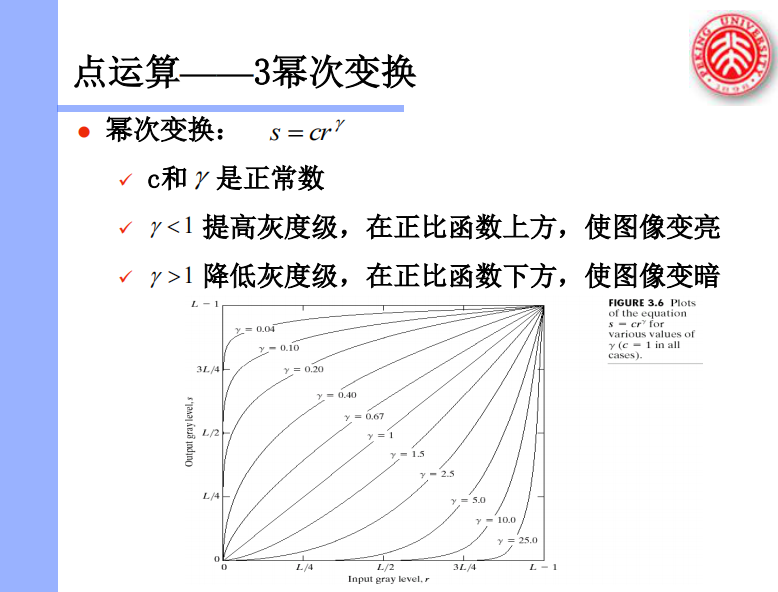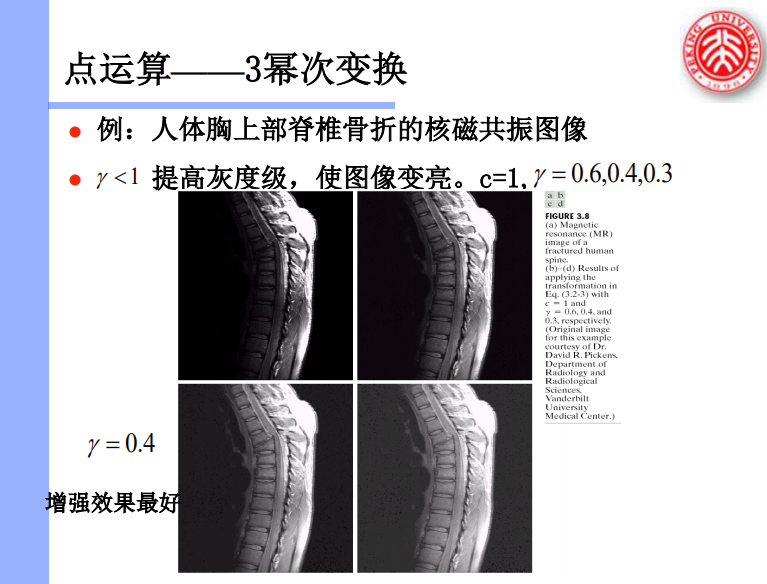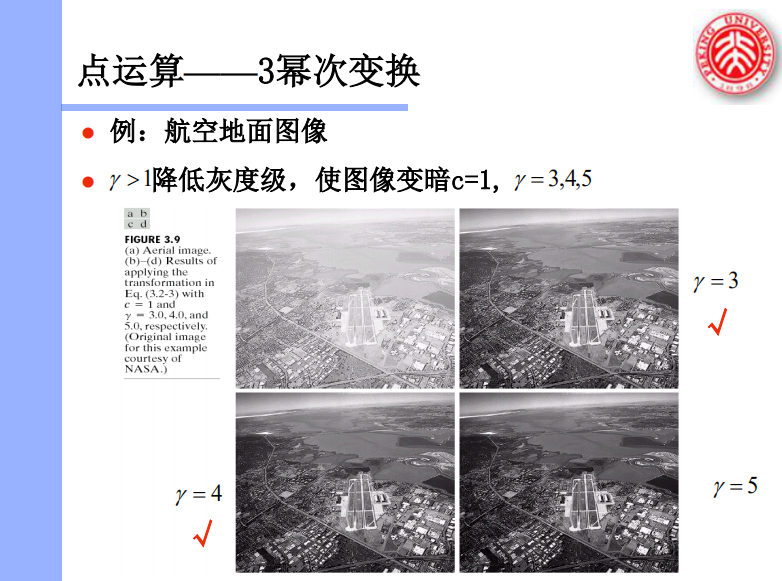• 代码实现时要注意原像素要先除以255.0进行归一化，幂次运算后再*255，否则会发生错误。

• 代码如下：

#include <string.h>
#include <math.h>
#include <stdio.h>
#include <stdlib.h>
#include <malloc.h>

#include<time.h>//时间相关头文件，可用其中函数计算图像处理速度

#define   WIDTHBYTES(bits) (((bits)+31)/32*4)//用于使图像宽度所占字节数为4byte的倍数

typedef unsigned char  BYTE;
typedef unsigned short WORD;
typedef unsigned long  DWORD;
typedef long LONG;

//位图文件头信息结构定义
//其中不包含文件类型信息（由于结构体的内存结构决定，要是加了的话将不能正确读取文件信息）

DWORD  bfSize;          //文件大小
WORD   bfReserved1;     //保留字，不考虑
WORD   bfReserved2;     //保留字，同上
DWORD  bfOffBits;       //实际位图数据的偏移字节数，即前三个部分长度之和

//public:
DWORD   biSize;             //指定此结构体的长度，为40
LONG    biWidth;            //位图宽
LONG    biHeight;           //位图高
WORD    biPlanes;           //平面数，为1
WORD    biBitCount;         //采用颜色位数，可以是1，2，4，8，16，24，新的可以是32
DWORD   biCompression;      //压缩方式，可以是0，1，2，其中0表示不压缩
DWORD   biSizeImage;        //实际位图数据占用的字节数
LONG    biXPelsPerMeter;    //X方向分辨率
LONG    biYPelsPerMeter;    //Y方向分辨率
DWORD   biClrUsed;          //使用的颜色数，如果为0，则表示默认值(2^颜色位数)
DWORD   biClrImportant;     //重要颜色数，如果为0，则表示所有颜色都是重要的

void main()
{
long now=0;
now=clock();//存储图像处理开始时间

FILE* pfile;
FILE* wfile;

char strFile="C:\\testpicture\\6.bmp";//打开图像路径，需修改为自己图像存储的路径
char strFilesave="C:\\testpicture\\8.bmp";//处理后图像存储路径，需修改为自己图像存储的路径
pfile = fopen(strFile,"rb");//文件打开图像
wfile = fopen(strFilesave,"wb");//打开文件为存储修改后图像做准备

//读取位图文件头信息
WORD fileType;
fwrite(&fileType,1,sizeof(WORD),wfile);
if(fileType != 0x4d42)
{
printf("file is not .bmp file!");
return;
}

//读取位图信息头信息

//分配内存空间把源图存入内存

BYTE    *pColorData=(BYTE *)malloc(height*l_width);//开辟内存空间存储图像数据
memset(pColorData,0,height*l_width);

BYTE    *pColorDataMid=(BYTE *)malloc(height*l_width);//开辟内存空间存储图像处理之后数据
memset(pColorDataMid,0,height*l_width);

long nData = height*l_width;

//把位图数据信息读到数组里
//在嵌入式开发环境下，大多数情况下已经得到图像数据区，是故将下面部分代码稍作修改就可以移植到嵌入式端

/*******************图像处理部分******************/
/*******************示例，将图像亮度减半******************/
for(int hnum=0;hnum<height;hnum++)
for(int wnum=0;wnum<width;wnum++)
{
int pixel_point=hnum*l_width+wnum*3;//数组位置偏移量，对应于图像的各像素点RGB的起点
pColorDataMid[pixel_point]=pow(pColorData[pixel_point]/ 255.0, 3) * 255;
pColorDataMid[pixel_point+1]=pow(pColorData[pixel_point+1]/ 255.0, 3) * 255;
pColorDataMid[pixel_point+2]=pow(pColorData[pixel_point+2]/ 255.0, 3) * 255;
}
/*******************示例，将图像亮度减半******************/
/*******************亮度控制******************/

fwrite(pColorDataMid,1,nData,wfile);//将处理完图像数据写回文件
fclose(pfile);
fclose(wfile);

printf("图像处理完成\n");
printf("运行时间为：%dms\n",clock()-now);//输出图像处理花费时间信息
}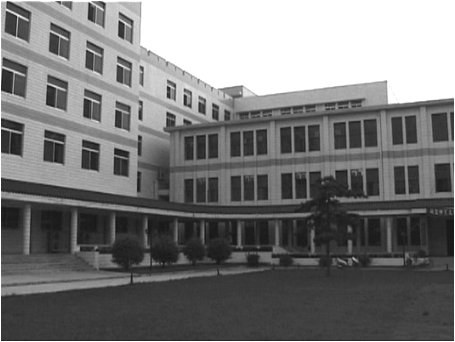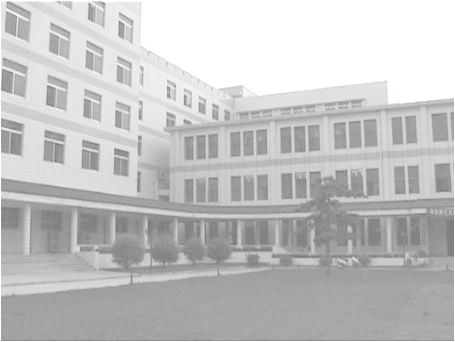C=1，γ=0.3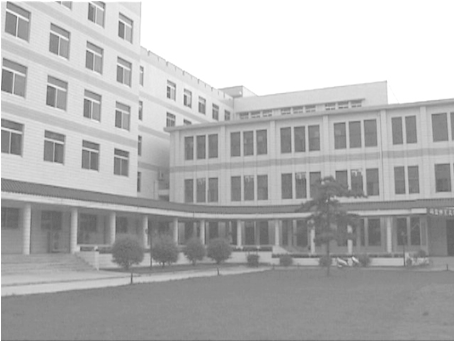C=1，γ=0.4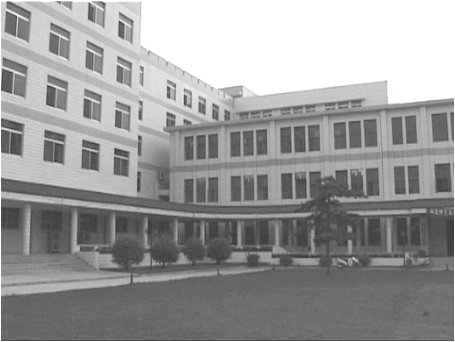C=1，γ=0.6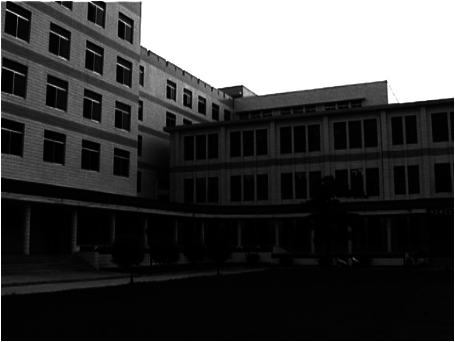C=1，γ=3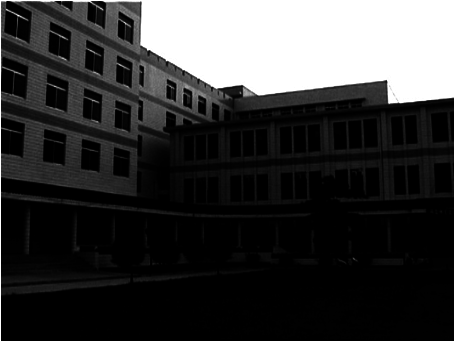C=1，γ=4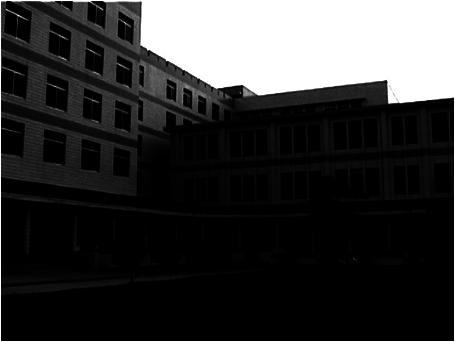C=1，γ=5

2019-09-08 18:15:18 qq_40664693 阅读数 453
• ###### MATLAB图像处理

全面系统的学习MATLAB在图像处理中的应用

19867 人正在学习 去看看 魏伟

1、因为需要找到图片中的四个圆，刚开始我是直接在整张图片上找，可是发现周围的干扰因素太多了，调了一组参数，发现重复的圆太多了，还有其他我们不需要的误圆检测了出来。然后就想着分别检测，为了摆脱周围相似环境的影响，我将整张图片带圆的地方，剪裁了下来，分别剪裁了只带圆的正方形进行检测，这样就比较容易检测，找到准确位置。（提示一点，可能分别检测的时候，也可能在同一个圆的边界附近检测出了很多相似圆重叠在一起，也就是检测同一个圆出来了好几组相近的坐标数据。你又无法调一组只出来一组坐标的参数，但是最后你只需要一组坐标数据，这时候你可以对矩阵进行求平均，用mean()函数即可。代码里有）
2、分别找到四个圆的准确位置后，也只是相对位置，再加上剪裁的起始坐标就得到了绝对坐标。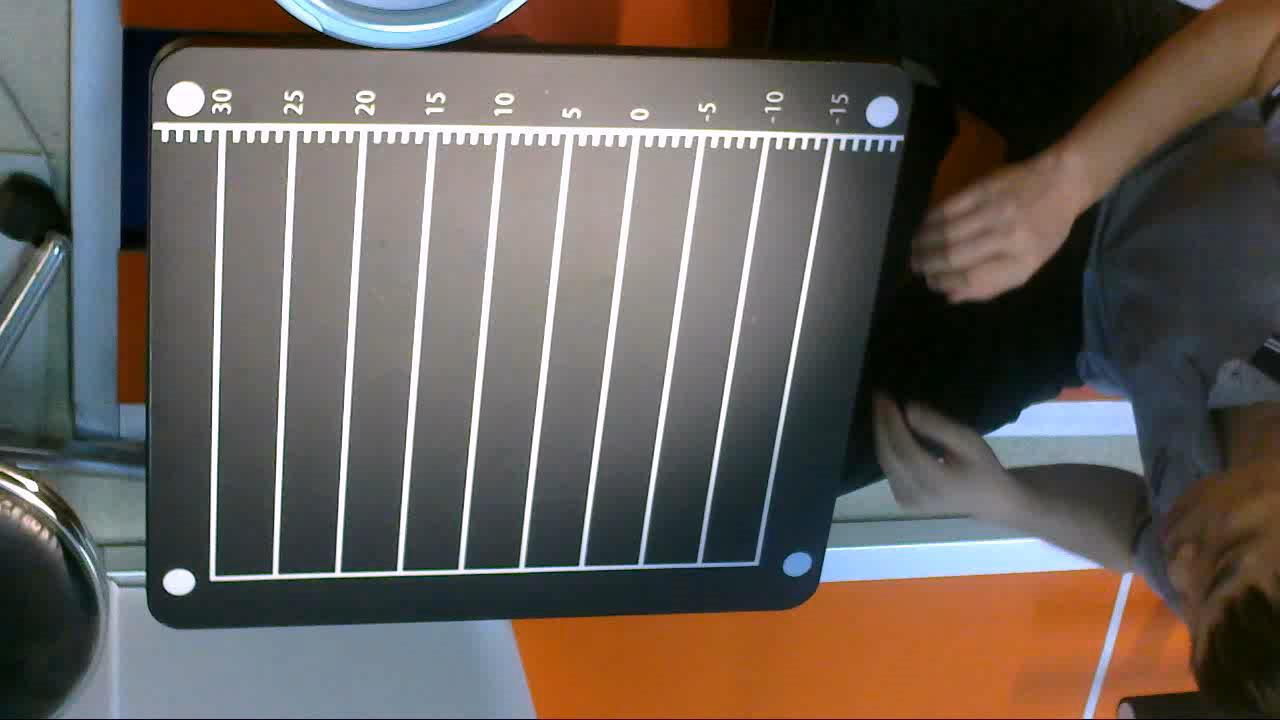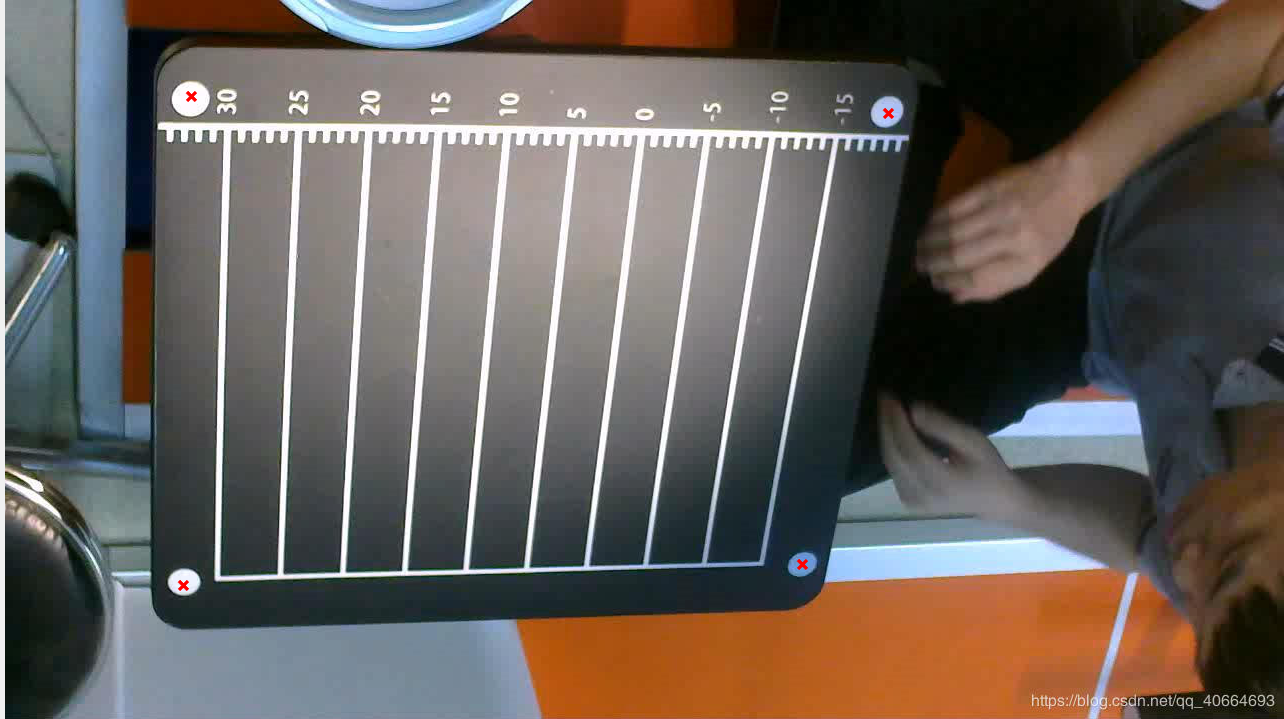main.m文件

%%     main.m文件
clc;
clear;
% BW :二值图像；
% stepR：检测的圆半径步长
% stepAngle：角度步长，单位为弧度
% minR：最小圆半径
% maxR：最大圆半径 p：阈值 0,1之间的数，通过调节此值可以得到图中圆的圆心和半径
% 读取视频的第一帧进行如下操作：
%1）剪裁图片的四个角
%2）利用霍夫变换检测圆，检测圆的之后得到圆的圆心并同时输出四个圆的坐标和半径。
%3）输出的有圆心的相对坐标和圆心的绝对坐标。
for i = 1:4 % 连续剪裁图片的四个角
switch (i)
case {1}
tlc= imcrop(I,[117 50 100 100]);% 左上角
processpic(tlc,1);
figure,imshow(tlc);
case {2}
dlc= imcrop(I,[115 520 100 100]);% 左下角
processpic(dlc,2);
figure,imshow(dlc);
case {3}
trc = imcrop(I,[810 45 100 100]); % 右上角
processpic(trc,3);
figure,imshow(trc);
case {4}
drc = imcrop(I,[745 510 100 100]); % 右下角
processpic(drc,4);
figure,imshow(drc);
end
end



processpic.m函数

对图片进行预处理
function processpic(t,h)
grayI = rgb2gray(t);%图片灰度处理
BW = edge(grayI,'sobel');%边缘检测
imshow(t);
hold on;
switch (h)
case {1}% 这是检测到的第一个圆
parm = findcircle(BW,3,0.07,16,18,0.95);
% 对于supple因为调参之后就出现了一组数据，所以不用再求均值。
%disp(parm);
%paraAvg = mean(parm);%对矩阵的每一列进行求均值
% 输出的坐标是绝对坐标坐标形式是（y,x）;
case {2}%这是检测到的第二个圆
parm = findcircle(BW,3,0.07,16,18,0.95);
% paraAvg = mean(parm);%对矩阵的每一列进行求均值
case {3}%这是检测的第三个圆
parm = findcircle(BW,3,0.07,16,18,0.95);
% paraAvg = mean(parm);%对矩阵的每一列进行求均值
case {4}%这是检测的第四个圆
parm = findcircle(BW,3,0.07,16,18,0.95);
% paraAvg = mean(parm);%对矩阵的每一列进行求均值
end

% 绘制所有的圆
for i = 1:size(parm,1)
x0 = parm(i,1); y0 = parm(i,2);  r0 = parm(i,3);
xi=[-r0:0 0:r0];
yi=round((r0^2-xi.^2).^0.5);
plot(yi+y0,xi+x0,'Color','g','LineWidth',3); % 下半圆
plot(-yi+y0,xi+x0,'Color','g','LineWidth',3); % 上半圆
% plot(y0,x0,'x','LineWidth',2,'Color','red');
end
end


findcircle.m函数

% 霍夫检测圆的主要代码
function [para] = findcircle(BW,stepR,stepAngle,minR,maxR,p)
%circleParaXYR = [];
[m,n] = size(BW);% BW:二值图像；
cntR = round((maxR-minR)/stepR)+1;% stepR:检测的圆半径步长， minR:最小圆半径， maxR:最大圆半径
cntAngle = round(2*pi/stepAngle);% stepAngle:角度步长，单位为弧度
hough_space = zeros(m,n,cntR);
% hough_space:参数空间，h(a,b,r)表示圆心在(a,b)半径为r的圆上的点数
[rows,cols] = find(BW);
cntPoints = size(rows,1);
% Hough变换将图像空间(x,y)对应到参数空间(a,b,r)
% a = x-r*cos(angle), b = y-r*sin(angle)
for i=1:cntPoints
for r=1:cntR
for k=1:cntAngle
a = round(rows(i)-(minR+(r-1)*stepR)*cos(k*stepAngle));
b = round(cols(i)-(minR+(r-1)*stepR)*sin(k*stepAngle));
if(a>0 && a<=m && b>0 && b<=n)
hough_space(a,b,r) = hough_space(a,b,r)+1;
end
end
end
end

% 寻找满足阈值的圆的参数
max_para = max(max(max(hough_space)));
index = find(hough_space>=max_para*p); % p:以p*hough_space的最大值为阈值，p取0，1之间的数
length = size(index,1);
hough_circle=zeros(m,n);
for i=1:cntPoints
for k=1:length
par3 = floor(index(k)/(m*n))+1;
par2 = floor((index(k)-(par3-1)*(m*n))/m)+1;
par1 = index(k)-(par3-1)*(m*n)-(par2-1)*m;
if((rows(i)-par1)^2+(cols(i)-par2)^2<(minR+(par3-1)*stepR)^2+5 && (rows(i)-par1)^2+(cols(i)-par2)^2>(minR+(par3-1)*stepR)^2-5)
hough_circle(rows(i),cols(i)) = 1;% hough_circl:二值图像，检测到的圆
end
end
end

for k=1:length
par3 = floor(index(k)/(m*n))+1;
par2 = floor((index(k)-(par3-1)*(m*n))/m)+1;    % 圆心y坐标
par1 = index(k)-(par3-1)*(m*n)-(par2-1)*m;      % 圆心x坐标
par3 = minR+(par3-1)*stepR;                    % 圆的半径
para(k,:) = [par1,par2,par3];% para:检测到的圆的圆心、半径
plot(par2,par1,'x','LineWidth',5,'Color','red');%用红的的X对圆心位置进行标记

end